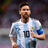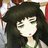首页   注册   登录
V2EX = way to explore
V2EX 是一个关于分享和探索的地方

Learn Python the Hard Way
Python 学习手册Python CookbookPython 基础教程Python Sites
PyPI - Python Package Index
http://www.simple-is-better.com/
http://diveintopython.org/toc/index.html
Pocoo

PyPy
Celery
Jinja2
gevent
pyenv
virtualenv
Stackless Python
Beautiful Soup

Green Unicorn
Sentry
Shovel
Pyflakes
pytest
Python 编程
pep8 Checker
Styles
PEP 8
Code Style from The Hitchhiker's Guide
V2EX  ›  Python

# sum() 函数性能堪忧，列表降维有何良方？（内有公号宣传，不喜勿进）

chinesehuazhou · 224 天前 · 1103 次点击Python 的内置函数 sum() 可以接收两个参数，当第一个参数是二维列表，第二个参数是一维列表的时候，它可以实现列表降维的效果。

`stackoverflow` 网站上，有人问了个“How to make a flat list out of list of lists”问题，正是我们在上篇文章中提出的问题。在回答中，有人分析了 7 种方法的时间性能。

``````import functools
import itertools
import numpy
import operator
import perfplot

def forfor(a):
return [item for sublist in a for item in sublist]

def sum_brackets(a):
return sum(a, [])

def functools_reduce(a):
return functools.reduce(operator.concat, a)

def functools_reduce_iconcat(a):
return functools.reduce(operator.iconcat, a, [])

def itertools_chain(a):
return list(itertools.chain.from_iterable(a))

def numpy_flat(a):
return list(numpy.array(a).flat)

def numpy_concatenate(a):
return list(numpy.concatenate(a))

perfplot.show(
setup=lambda n: [list(range(10))] * n,
kernels=[
forfor, sum_brackets, functools_reduce, functools_reduce_iconcat,
itertools_chain, numpy_flat, numpy_concatenate
],
n_range=[2**k for k in range(16)],
logx=True,
logy=True,
xlabel='num lists'
)
````````````from itertools import chain
from functools import reduce
from collections import Iterable  # or from collections.abc import Iterable
import operator
from iteration_utilities import deepflatten

def nested_list_comprehension(lsts):
return [item for sublist in lsts for item in sublist]

def itertools_chain_from_iterable(lsts):
return list(chain.from_iterable(lsts))

def pythons_sum(lsts):
return sum(lsts, [])

return reduce(lambda x, y: x + y, lsts)

def pylangs_flatten(lsts):
return list(flatten(lsts))

def flatten(items):
"""Yield items from any nested iterable; see REF."""
for x in items:
if isinstance(x, Iterable) and not isinstance(x, (str, bytes)):
yield from flatten(x)
else:
yield x

def reduce_concat(lsts):
return reduce(operator.concat, lsts)

def iteration_utilities_deepflatten(lsts):
return list(deepflatten(lsts, depth=1))

from simple_benchmark import benchmark

b = benchmark(
pylangs_flatten, reduce_concat, iteration_utilities_deepflatten],
arguments={2**i: [*5]*(2**i) for i in range(1, 13)},
argument_name='number of inner lists'
)

b.plot()
````````````/* It's tempting to use PyNumber_InPlaceAdd instead of
when doing 'sum(list_of_lists, [])'.  However, this
would produce a change in behaviour: a snippet like

empty = []
sum([[x] for x in range(10)], empty)

would change the value of empty. */
``````

``````operator.iconcat(a, b)
operator.__iconcat__(a, b)
a = iconcat(a, b) is equivalent to a += b for a and b sequences.
``````2 回复1 iorilu   224 天前 via iPhone 不错，还不知道 sum 可以有两个参数2 chenstack   224 天前 sum 是在 start 参数基础上在元素上调用__add__，如果元素是 list，每次 add 都生成一个新的 list 对象，性能肯定差这个场景下 operator.iadd 应该和 operator.iconcat 差不多